# ML Aggarwal Class 10 Solutions for ICSE Maths Chapter 6 Factorization Ex 6

## ML Aggarwal Class 10 Solutions for ICSE Maths Chapter 6 Factorization Ex 6

ML Aggarwal Class 10 Solutions for ICSE Maths Chapter 6 Factorization Ex 6

Question 1.
Find the remainder (without divisions) on dividing f(x) by x – 2, where
(i) f(x) = 5x2 – 1x + 4
(ii) f(x) = 2x3 – 7x2 + 3
Solution:Question 2.
Using remainder theorem, find the remainder on dividing f(x) by (x + 3) where
(i) f(x) = 2x2 – 5x + 1
(ii) f(x) = 3x3 + 7x2 – 5x + 1
Solution:Question 3.
Find the remainder (without division) on dividing f(x) by (2x + 1) where
(i) f(x) = 4x2 + 5x + 3
(ii) f(x) = 3x3 – 7x2 + 4x + 11
Solution:Question 4.
(i) Find the remainder (without division) when 2x3 – 3x2 + 7x – 8 is divided by x – 1 (2000)
(ii) Find the remainder (without division) on dividing 3x2 + 5x – 9 by (3x + 2)
Solution: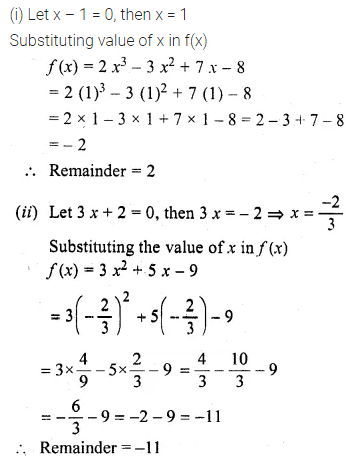Question 5.
Using remainder theorem, find the value of k if on dividing 2x3 + 3x2 – kx + 5 by x – 2, leaves a remainder 7. (2016)
Solution:Question 6.
Using remainder theorem, find the value of a, if the division of x3 + 5x2 – ax + 6 by (x – 1) leaves the remainder 2a.
Solution: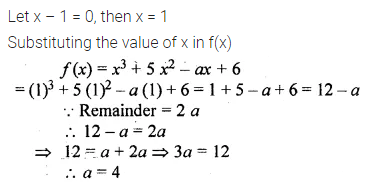Question 7.
(i) What number must be subtracted from 2x2 – 5x so that the resulting polynomial leaves the remainder 2 when divided by 2x + 1?
(ii) What number must be added to 2x3 – 7x2 + 2x so that the resulting polynomial leaves the remainder -2 when divided by 2x – 3?
Solution: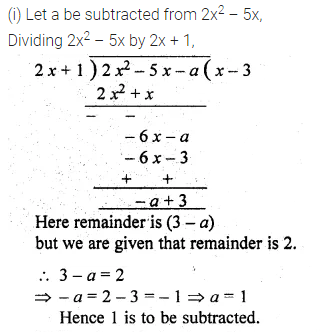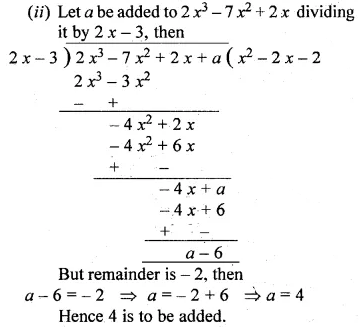Question 8.
(i) When divided by x – 3 the polynomials x2 – px2 + x + 6 and 2x3 – x2 – (p + 3) x – 6 leave the same remainder. Find the value of ‘p’
(ii) Find ‘a’ if the two polynomials ax3 + 3x2 – 9 and 2x3 + 4x + a, leaves the same remainder when divided by x + 3.
Solution: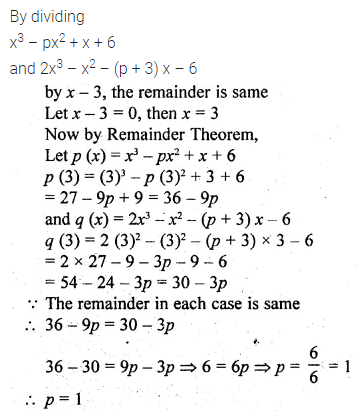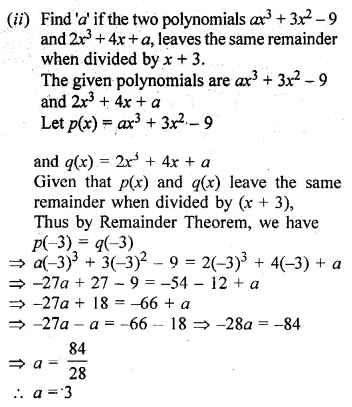Question 9.
By factor theorem, show that (x + 3) and (2x – 1) are factors of 2x2 + 5x – 3.
Solution:Question 10.
Show that (x – 2) is a factor of 3x2 – x – 10. Hence factorise 3x2 – x – 10.
Solution: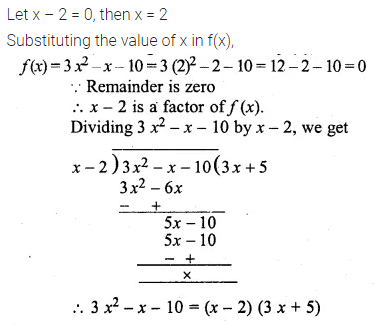Question 11.
Show that (x – 1) is a factor of x3 – 5x2 – x + 5 Hence factorise x3 – 5x2 – x + 5.
Solution:Question 12.
Show that (x – 3) is a factor of x3 – 7x2 + 15x – 9. Hence factorise x3 – 7x2 + 15 x – 9
Solution:Question 13.
Show that (2x + 1) is a factor of 4x3 + 12x2 + 11 x + 3. Hence factorise 4x3 + 12x2 + 11x + 3.
Solution: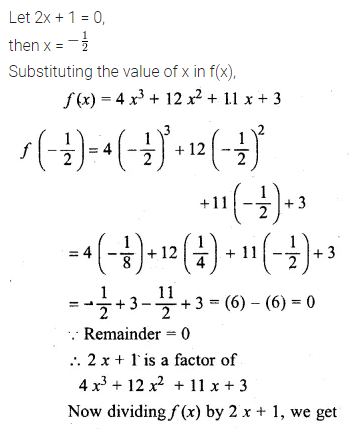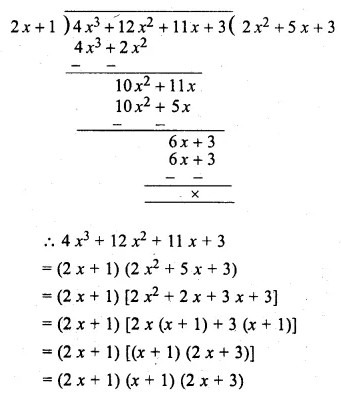Question 14.
Show that 2x + 7 is a factor of 2x3 + 5x2 – 11x – 14. Hence factorize the given expression completely, using the factor theorem. (2006)
Solution: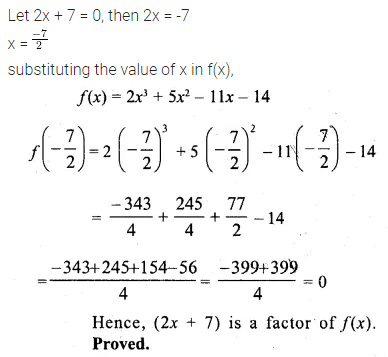Question 15.
Use factor theorem to factorise the following polynominals completely.
(i) x3 + 2x2 – 5x – 6
(ii) x3 – 13x – 12.
Solution: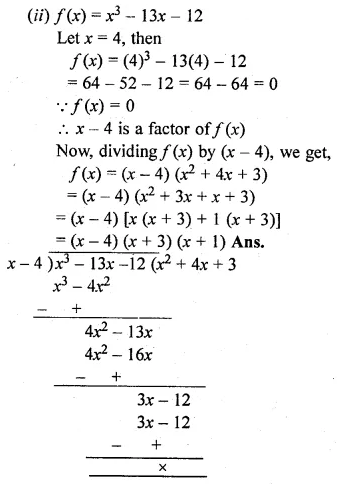Question 16.
(i) Use the Remainder Theorem to factorise the following expression : 2x3 + x2 – 13x + 6. (2010)
(ii) Using the Remainder Theorem, factorise completely the following polynomial: 3x2 + 2x2 – 19x + 6 (2012)
Solution: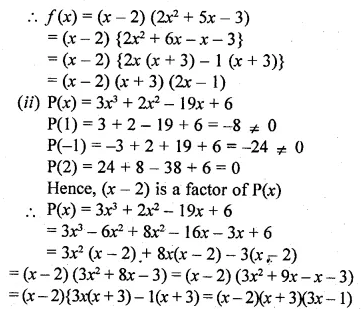Question 17.
Using the Remainder and Factor Theorem, factorize the following polynomial: x3 + 10x2 – 37x + 26.
Solution:Question 18.
If (2x + 1) is a factor of 6x3 + 5x2 + ax – 2 find the value of a
Solution:Question 19.
If (3x – 2) is a factor of 3x3 – kx2 + 21x – 10, find the value of k.
Solution: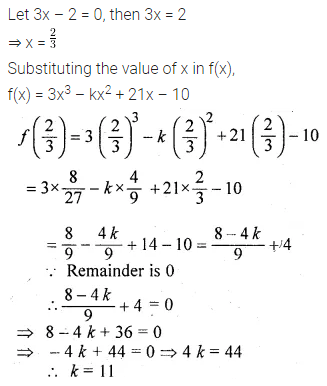Question 20.
If (x – 2) is a factor of 2x3 – x2 + px – 2, then
(i) find the value of p.
(ii) with this value of p, factorize the above expression completely
Solution:Question 21.
Find the value of ‘K’ for which x = 3 is a solution of the quadratic equation, (K + 2)x2 – Kx + 6 = 0.
Also, find the other root of the equation.
Solution:Question 22.
What number should be subtracted from 2x3 – 5x2 + 5x so that the resulting polynomial has 2x – 3 as a factor?
Solution: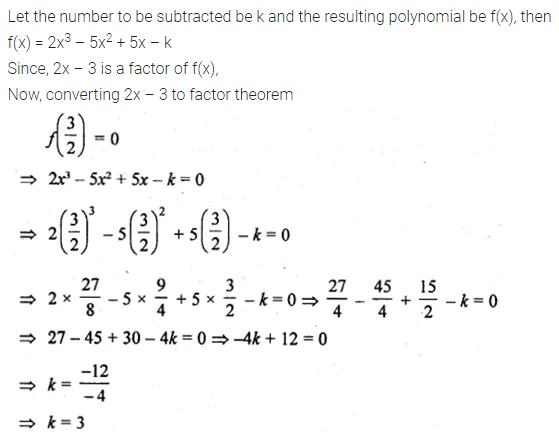Question 23.
Find the value of the constants a and b, if (x – 2) and (x + 3) are both factors of the expression x3 + ax2 + bx – 12.
Solution:Question 24.
If (x + 2) and (x – 3) are factors of x3 + ax + b, find the values of a and b. With these values of a and b, factorize the given expression.
Solution: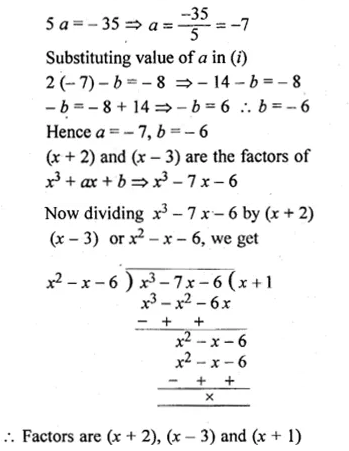Question 25.
(x – 2) is a factor of the expression x3 + ax2 + bx + 6. When this expression is divided by (x – 3), it leaves the remainder 3. Find the values of a and b. (2005)
Solution: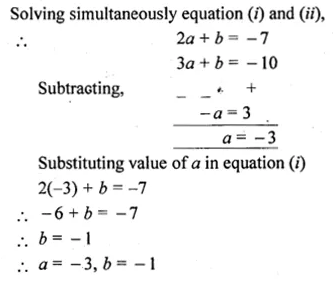Question 26.
If (x – 2) is a factor of the expression 2x3 + ax2 + bx – 14 and when the expression is divided by (x – 3), it leaves a remainder 52, find the values of a and b.
Solution: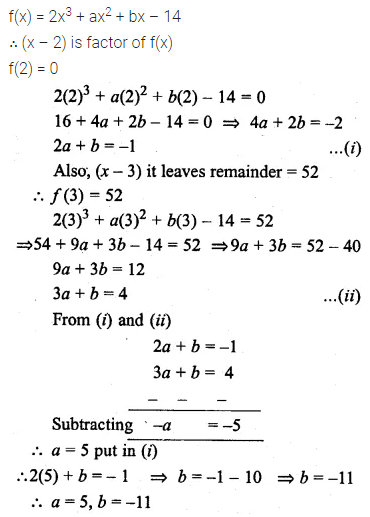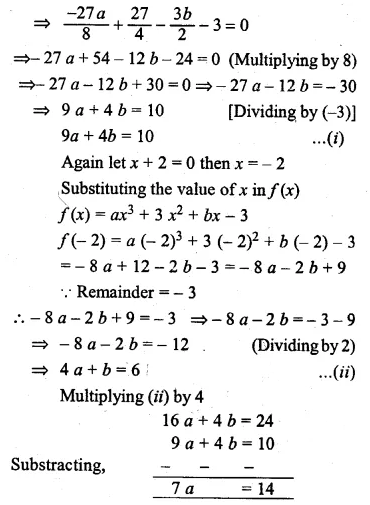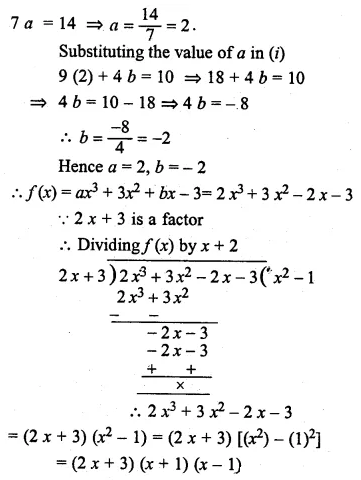Question 27.
If ax3 + 3x2 + bx – 3 has a factor (2x + 3) and leaves remainder – 3 when divided by (x + 2), find the values of a and 6. With these values of a and 6, factorize the given expression.
Solution:Question 28.
Given f(x) = ax2 + bx + 2 and g(x) = bx2 + ax + 1. If x – 2 is a factor of f(x) but leaves the remainder – 15 when it divides g(x), find the values of a and b. With these values of a and b, factorise the expression. f(x) + g(x) + 4x2 + 7x.
Solution: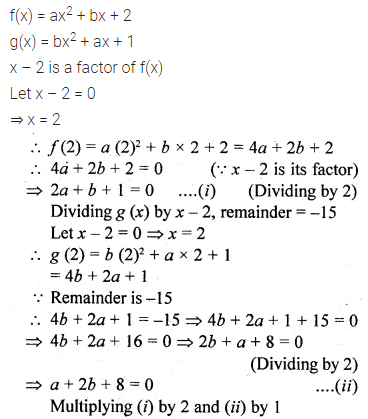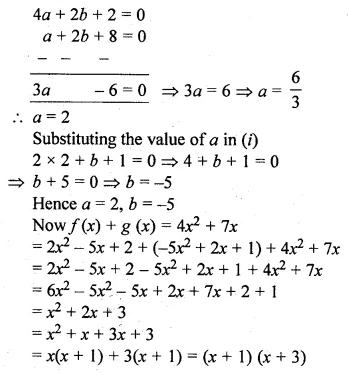ML Aggarwal Class 10 Solutions for ICSE Maths